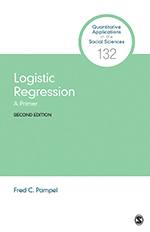# Logistic Regression: A Primer

This volume helps readers understand the intuitive logic behind logistic regression through nontechnical language and simple examples. The Second Edition presents results from several statistical packages to help interpret the meaning of logistic regression coefficients, presents more detail on variations in logistic regression for multicategory outcomes, and describes some potential problems in interpreting logistic regression coefficients. A companion website includes the three data sets and Stata, SPSS, and R commands needed to reproduce all the tables and figures in the book. Finally, the Appendix reviews the meaning of logarithms, and helps readers understand the use of logarithms in logistic regression as well as in other types of models.

•••